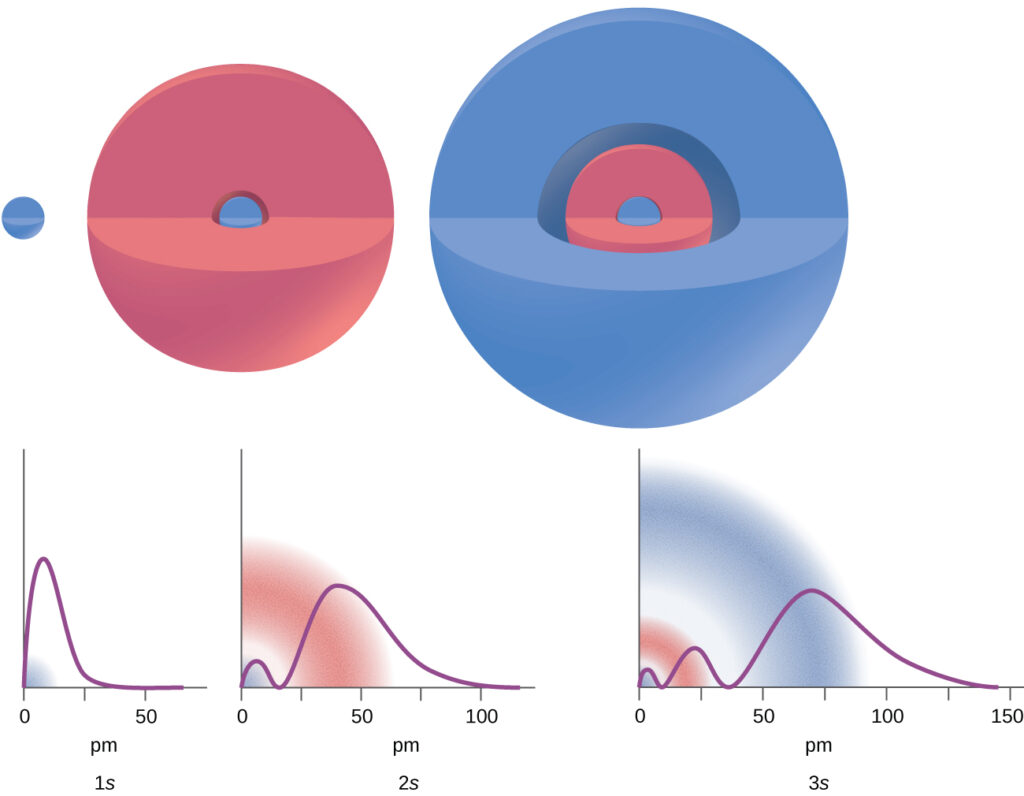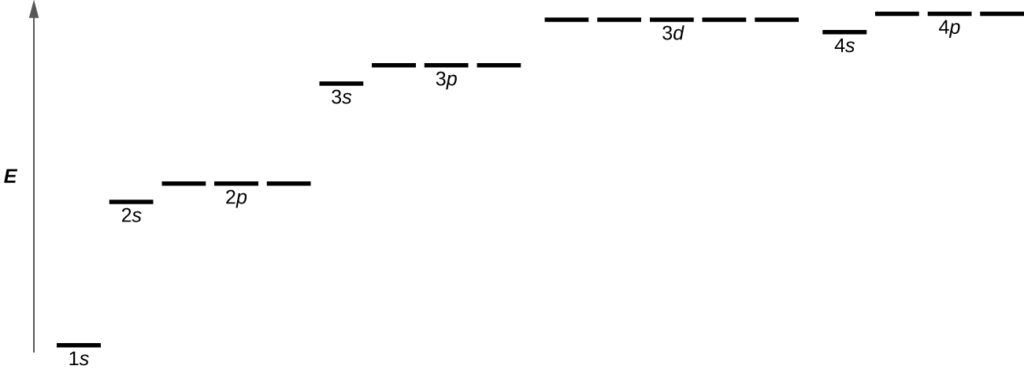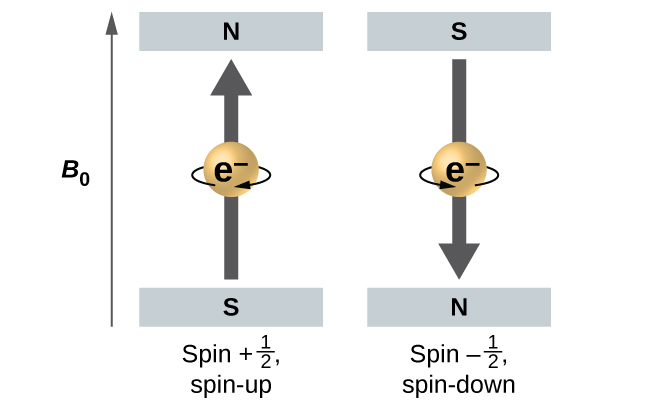The goal of this section is to understand the electron orbitals (location of electrons in atoms), their different energies, and other properties. The use of quantum theory provides the best understanding to these topics. This knowledge is a precursor to chemical bonding.

As was described previously, electrons in atoms can exist only on discrete energy levels but not between them. It is said that the energy of an electron in an atom is quantized, that is, it can be equal only to certain specific values and can jump from one energy level to another but not transition smoothly or stay between these levels.

The energy levels are labeled with an n value, where n = 1, 2, 3, …. Generally speaking, the energy of an electron in an atom is greater for greater values of n. This number, n, is referred to as the principal quantum number. The principal quantum number defines the location of the energy level. It is essentially the same concept as the n in the Bohr atom description. Another name for the principal quantum number is the shell number. The shells of an atom can be thought of concentric circles radiating out from the nucleus. The electrons that belong to a specific shell are most likely to be found within the corresponding circular area. The further we proceed from the nucleus, the higher the shell number, and so the higher the energy level ([link]). The positively charged protons in the nucleus stabilize the electronic orbitals by electrostatic attraction between the positive charges of the protons and the negative charges of the electrons. So the further away the electron is from the nucleus, the greater the energy it has.

This quantum mechanical model for where electrons reside in an atom can be used to look at electronic transitions, the events when an electron moves from one energy level to another. If the transition is to a higher energy level, energy is absorbed, and the energy change has a positive value. To obtain the amount of energy necessary for the transition to a higher energy level, a photon is absorbed by the atom. A transition to a lower energy level involves a release of energy, and the energy change is negative. This process is accompanied by emission of a photon by the atom. The following equation summarizes these relationships and is based on the hydrogen atom:

$$ΔE=E_{final}−E_{initial}$$

$$ΔE =−2.18×10^{−18}(\frac{1}{n_f^2}−\frac{1}{n_i^2})\;J$$

The values nf and ni are the final and initial energy states of the electron. [link] in the previous section of the chapter demonstrates calculations of such energy changes.

The principal quantum number is one of three quantum numbers used to characterize an orbital. An atomic orbital is a general region in an atom within which an electron is most probable to reside. The quantum mechanical model specifies the probability of finding an electron in the three-dimensional space around the nucleus and is based on solutions of the Schrödinger equation. In addition, the principal quantum number defines the energy of an electron in a hydrogen or hydrogen-like atom or an ion (an atom or an ion with only one electron) and the general region in which discrete energy levels of electrons in a multi-electron atoms and ions are located.

Another quantum number is l, the secondary (angular momentum) quantum number. It is an integer that may take the values, l = 0, 1, 2, …, n – 1. This means that an orbital with n = 1 can have only one value of l, l = 0, whereas n = 2 permits l = 0 and l = 1, and so on. Whereas the principal quantum number, n, defines the general size and energy of the orbital, the secondary quantum number l specifies the shape of the orbital. Orbitals with the same value of l define a subshell.

Orbitals with l = 0 are called s orbitals and they make up the s subshells. The value l = 1 corresponds to the p orbitals. For a given n, p orbitals constitute a p subshell (e.g., 3p if n = 3). The orbitals with l = 2 are called the d orbitals, followed by the f-, g-, and h-orbitals for l = 3, 4, and 5.

There are certain distances from the nucleus at which the probability density of finding an electron located at a particular orbital is zero. In other words, the value of the wavefunction ψ is zero at this distance for this orbital. Such a value of radius r is called a radial node. The number of radial nodes in an orbital is nl – 1.The graphs show the probability (y axis) of finding an electron for the 1s, 2s, 3s orbitals as a function of distance from the nucleus.

Consider the examples in [link]. The orbitals depicted are of the s type, thus l = 0 for all of them. It can be seen from the graphs of the probability densities that there are 1 – 0 – 1 = 0 places where the density is zero (nodes) for 1s (n = 1), 2 – 0 – 1 = 1 node for 2s, and 3 – 0 – 1 = 2 nodes for the 3s orbitals.

The s subshell electron density distribution is spherical and the p subshell has a dumbbell shape. The d and f orbitals are more complex. These shapes represent the three-dimensional regions within which the electron is likely to be found.

The magnetic quantum number, ml, specifies the relative spatial orientation of a particular orbital. Generally speaking, ml can be equal to –l, –(l – 1), …, 0, …, (l – 1), l. The total number of possible orbitals with the same value of l (that is, in the same subshell) is 2l + 1. Thus, there is one s-orbital in an s subshell (l = 0), there are three p-orbitals in a p subshell (l = 1), five d-orbitals in a d subshell (l = 2), seven f-orbitals in an f subshell (l = 3), and so forth. The principal quantum number defines the general value of the electronic energy. The angular momentum quantum number determines the shape of the orbital. And the magnetic quantum number specifies orientation of the orbital in space, as can be seen in [link].The chart shows the energies of electron orbitals in a multi-electron atom.

[link] illustrates the energy levels for various orbitals. The number before the orbital name (such as 2s, 3p, and so forth) stands for the principal quantum number, n. The letter in the orbital name defines the subshell with a specific angular momentum quantum number l = 0 for s orbitals, 1 for p orbitals, 2 for d orbitals. Finally, there are more than one possible orbitals for l ≥ 1, each corresponding to a specific value of ml. In the case of a hydrogen atom or a one-electron ion (such as He+, Li2+, and so on), energies of all the orbitals with the same n are the same. This is called a degeneracy, and the energy levels for the same principal quantum number, n, are called degenerate orbitals. However, in atoms with more than one electron, this degeneracy is eliminated by the electron–electron interactions, and orbitals that belong to different subshells have different energies, as shown on [link]. Orbitals within the same subshell are still degenerate and have the same energy.

While the three quantum numbers discussed in the previous paragraphs work well for describing electron orbitals, some experiments showed that they were not sufficient to explain all observed results. It was demonstrated in the 1920s that when hydrogen-line spectra are examined at extremely high resolution, some lines are actually not single peaks but, rather, pairs of closely spaced lines. This is the so-called fine structure of the spectrum, and it implies that there are additional small differences in energies of electrons even when they are located in the same orbital. These observations led Samuel Goudsmit and George Uhlenbeck to propose that electrons have a fourth quantum number. They called this the spin quantum number, or ms.

The other three quantum numbers, n, l, and ml, are properties of specific atomic orbitals that also define in what part of the space an electron is most likely to be located. Orbitals are a result of solving the Schrödinger equation for electrons in atoms. The electron spin is a different kind of property. It is a completely quantum phenomenon with no analogues in the classical realm. In addition, it cannot be derived from solving the Schrödinger equation and is not related to the normal spatial coordinates (such as the Cartesian x, y, and z). Electron spin describes an intrinsic electron “rotation” or “spinning.” Each electron acts as a tiny magnet or a tiny rotating object with an angular momentum, or as a loop with an electric current, even though this rotation or current cannot be observed in terms of spatial coordinates.

The magnitude of the overall electron spin can only have one value, and an electron can only “spin” in one of two quantized states. One is termed the α state, with the $z$ component of the spin being in the positive direction of the $z$ axis. This corresponds to the spin quantum number $m_s​=\frac{1}{2}$. The other is called the β state, with the z component of the spin being negative and $m_s​=-\frac{1}{2}$. Any electron, regardless of the atomic orbital it is located in, can only have one of those two values of the spin quantum number. The energies of electrons having $m_s​=-\frac{1}{2}$ and $m_s​=\frac{1}{2}$ are different if an external magnetic field is applied.Electrons with spin values   ±12±12 in an external magnetic field.

[link] illustrates this phenomenon. An electron acts like a tiny magnet. Its moment is directed up (in the positive direction of the z axis) for the $\frac{1}{2}$ spin quantum number and down (in the negative z direction) for the spin quantum number of $-\frac{1}{2}$. A magnet has a lower energy if its magnetic moment is aligned with the external magnetic field (the left electron on [link]) and a higher energy for the magnetic moment being opposite to the applied field. This is why an electron with $m_s=\frac{1}{2}$ has a slightly lower energy in an external field in the positive z direction, and an electron with $m_s=-\frac{1}{2}$ has a slightly higher energy in the same field. This is true even for an electron occupying the same orbital in an atom. A spectral line corresponding to a transition for electrons from the same orbital but with different spin quantum numbers has two possible values of energy; thus, the line in the spectrum will show a fine structure splitting.